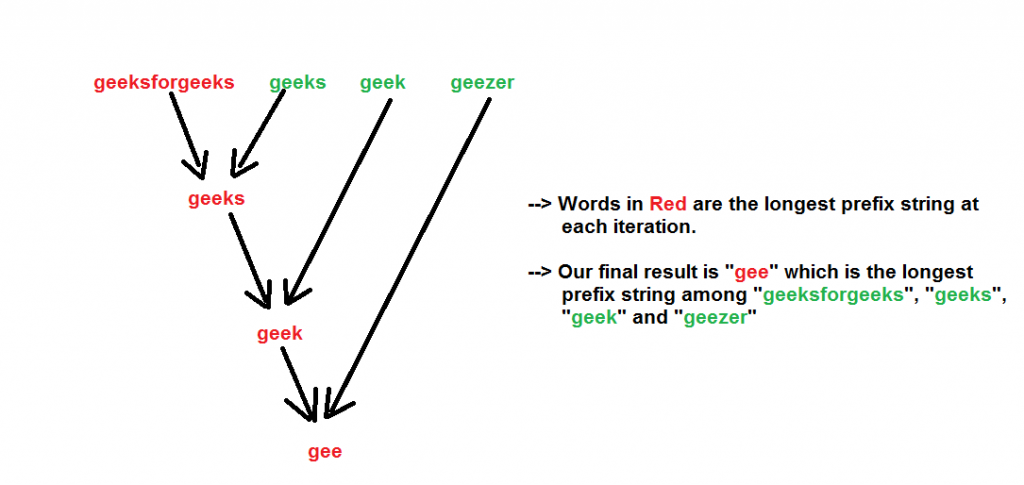# Java Program To Find Longest Common Prefix Using Word By Word Matching

• Last Updated : 03 Jan, 2022

Given a set of strings, find the longest common prefix.
Examples:

```Input  : {“geeksforgeeks”, “geeks”, “geek”, “geezer”}
Output : "gee"

Input  : {"apple", "ape", "april"}
Output : "ap"```

We start with an example. Suppose there are two strings- “geeksforgeeks” and “geeks”. What is the longest common prefix in both of them? It is “geeks”.
Now let us introduce another word “geek”. So now what is the longest common prefix in these three words ? It is “geek”
We can see that the longest common prefix holds the associative property, i.e-

```LCP(string1, string2, string3)
= LCP (LCP (string1, string2), string3)

Like here

LCP (“geeksforgeeks”, “geeks”, “geek”)
=  LCP (LCP (“geeksforgeeks”, “geeks”), “geek”)
=  LCP (“geeks”, “geek”) = “geek”```

So we can make use of the above associative property to find the LCP of the given strings. We one by one calculate the LCP of each of the given string with the LCP so far. The final result will be our longest common prefix of all the strings.
Note that it is possible that the given strings have no common prefix. This happens when the first character of all the strings are not same.
We show the algorithm with the input strings- “geeksforgeeks”, “geeks”, “geek”, “geezer” by the below figure.Below is the implementation of above approach:

## Java

 `// Java Program to find the longest  ` `// common prefix  ` `class` `GFG { ` ` `  `// A Utility Function to find the common  ` `// prefix between strings- str1 and str2  ` `static` `String commonPrefixUtil(String str1,  ` `                               ``String str2)  ` `{ ` `    ``String result = ``""``; ` `    ``int` `n1 = str1.length(),  ` `        ``n2 = str2.length(); ` ` `  `    ``// Compare str1 and str2  ` `    ``for` `(``int` `i = ``0``, j = ``0``; i <= n1 - ``1` `&&  ` `             ``j <= n2 - ``1``; i++, j++)  ` `    ``{ ` `        ``if` `(str1.charAt(i) != str2.charAt(j))  ` `        ``{ ` `            ``break``; ` `        ``} ` `            ``result += str1.charAt(i); ` `    ``} ` `        ``return` `(result); ` `} ` ` `  `// A Function that returns the longest  ` `// common prefix from the array of strings  ` `static` `String commonPrefix(String arr[],  ` `                           ``int` `n)  ` `{ ` `    ``String prefix = arr[``0``]; ` ` `  `    ``for` `(``int` `i = ``1``; i <= n - ``1``; i++)  ` `    ``{ ` `        ``prefix = commonPrefixUtil(prefix, arr[i]); ` `    ``} ` `        ``return` `(prefix); ` `} ` ` `  `// Driver code ` `public` `static` `void` `main(String[] args)  ` `{ ` `    ``String arr[] = {``"geeksforgeeks"``, ``"geeks"``, ` `                    ``"geek"``, ``"geezer"``}; ` `    ``int` `n = arr.length; ` `    ``String ans = commonPrefix(arr, n); ` ` `  `    ``if` `(ans.length() > ``0``)  ` `    ``{ ` `        ``System.out.printf(``"The longest common prefix is - %s"``, ` `                           ``ans); ` `    ``}  ` `    ``else`  `    ``{ ` `        ``System.out.printf(``"There is no common prefix"``); ` `    ``} ` `} ` `} ` `// This code is contributed by 29AjayKumar `

Output :

`The longest common prefix is - gee`

Time Complexity : Since we are iterating through all the strings and for each string we are iterating though each characters, so we can say that the time complexity is O(N M) where,

```N = Number of strings
M = Length of the largest string string ```

Auxiliary Space : To store the longest prefix string we are allocating space which is O(M).
Please refer complete article on Longest Common Prefix using Word by Word Matching for more details!

My Personal Notes arrow_drop_up
Recommended Articles
Page :